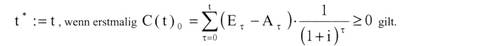# What is dynamic discounting

## Dynamic amortization calculation

The dynamic amortization calculation is based on the fact that the net present value of an investment (net present value method) usually increases over time, all other things being equal. The growth of the capital value C0 takes place with decreasing growth rates, since the cash value returns (net payments) of an extension year are smaller, the further the extension year is in the future.

The dynamic amortization time td of an investment is the time at which the net present value of the investment in question is just zero.

Or:
The dynamic amortization time of an investment is the time that elapses until the investor has recovered the purchase price plus interest.

An investment is advantageous in terms of the dynamic amortization criterion if its actual dynamic amortization time td is not greater than the maximum permissible amortization time tmax.

According to this, those investments are advantageous which, within their useful life of n years, guarantee both the recovery of the purchase price and the interest on the amounts still outstanding in the property.

Example:
We are looking for a static and dynamic amortization period for an investment with:

Purchase payment 100,000 DM
Lifespan 10 years
Discount rate 10%
constant annual
Returns 20,000 DM
Residual value 0 DM

The static amortization period (amortization calculation) is t = 5 years. After this time, the purchase price is recovered without interest. The system only amortized dynamically after td = 7.3 years. Thereafter, the purchase payment plus interest on the outstanding amounts is recovered.

Problem:
(1) The investor must be able to estimate the payment parameters characterizing the investment and the useful life with sufficient accuracy.

(2) He must determine his discount rate and the specified maximum amortization period (both subjective values) in a meaningful way.

(3) The? Dynamic amortization period? is only an aid to decision-making. If possible, it should be supplemented by further dynamic indicators (capital value, internal interest rate, average annual surplus) in order to achieve a well-rounded picture of the investment.

The amortization calculation (also PayOffRechnung) is a method for assessing the profitability of investment projects. The assessment criterion is the amortization time (PayOff time), i.e. the time that is required until the purchase costs of an investment alternative are covered by the return flows. A single investment project is considered beneficial if the payback period does not exceed a specified maximum time. If there are several investment alternatives to choose from, the one with the shortest payback period is considered to be the most advantageous. In contrast to the other methods of investment calculation, which assess the investment objects directly or indirectly according to the goal of profit maximization, the aim of the amortization calculation is to minimize risk. It is based on the idea that forecasts are more uncertain the further the forecasted data are in the future. As a result, the recovery of the capital employed is all the more certain, the shorter the payback period. However, the amortization period represents a very rough risk measure. Due to this assessment criterion, the amortization calculation cannot provide any information about the profitability of an investment alternative. It is entirely possible that an alternative with the higher profitability will have the longer payback period. With the dynamic amortization calculation, the amortization time t is determined for the simple so-called accumulation calculation according to the formula t a0 Z Rt 0 t = l with a0 = purchase expenditure, Rt = return flow of the year t. From this, t is to be determined by adding up the forecast returns per period over so many periods until their total reaches the purchase expenditure. The return flow of capital is calculated as the excess payment. It can also be expressed as the sum of profit, imputed depreciation and that part of imputed interest that exceeds borrowing interest. In order to include the different temporal structures of the returns in the calculation, the returns can be discounted. The amortization time t is then determined according to the formula t a0Z R, q "’ = 0. t1 59. The amortization period, taking interest into account, is always longer than that without interest. It indicates the point in time at which the net present value of the investment becomes positive.

see amortization calculation, dynamic (investment calculation).

(in the investment calculation). The dynamic amortization calculation is one of the classic partial models of the dynamic investment calculation (investment calculations, dynamic). The determination of the amortization period t * (other terms: amortization time, capital recovery time, capital return time, payback time) is based on the calculation rules of the net present value. The inpayments Et and disbursements At occurring in the lapse of time t are discounted with the discount rate i and added up until the capital value C (t) 0 is greater than or equal to zero for the first time. Formally it results:An individual investment assessed according to this criterion is advantageous if its amortization period is less than a specified limit value. When deciding between several investments, the one that leads to the shortest payback period should be chosen. See also investment calculations (investment decisions), static or dynamic or under uncertainty as well as investment management, each with references to the literature.

Previous technical term: Dynamic protection | Next technical term: Dynamic payback period

Report this article to the editors as incorrect and reserve it for editing